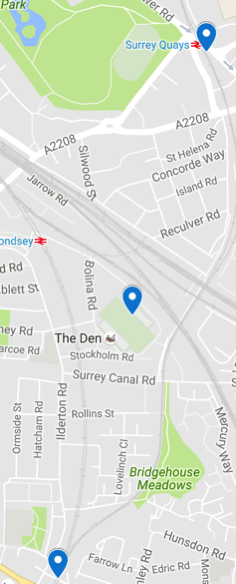# Neo4j: Find the midpoint between two lat/longsOver the last couple of weekends I’ve been playing around with some transport data and I wanted to run the A* algorithm to find the quickest route between two stations.

The A* algorithm takes an estimateEvaluator as one of its parameters and the evaluator looks at lat/longs of nodes to work out whether a path is worth following or not. I therefore needed to add lat/longs for each station and I found it surprisingly hard to find this location date for all the points in the dataset.

Luckily I tend to have the lat/longs for two points either side of a station so I can work out the midpoint as an approximation for the missing one.

I found an article which defines a formula we can use to do this and there’s a StackOverflow post which has some Java code that implements the formula.

I wanted to find the midpoint between Surrey Quays station (51.4931963543,-0.0475185810) and a point further south on the train line (51.47908,-0.05393950). I wrote the following Cypher query to calculate this point:

``````WITH 51.4931963543 AS lat1, -0.0475185810 AS lon1,
51.47908 AS lat2 , -0.05393950 AS lon2

WITH rlat1, rlon1, rlat2, rlon2,
cos(rlat2) * cos(dLon) AS Bx,
cos(rlat2) * sin(dLon) AS By

WITH atan2(sin(rlat1) + sin(rlat2),
sqrt( (cos(rlat1) + Bx) * (cos(rlat1) + Bx) + By * By )) AS lat3,
rlon1 + atan2(By, cos(rlat1) + Bx) AS lon3

RETURN degrees(lat3) AS midLat, degrees(lon3) AS midLon``````
``````╒═════════════════╤═════════════════════╕
│midLat           │midLon               │
╞═════════════════╪═════════════════════╡
│51.48613822097523│-0.050729537454086385│
└─────────────────┴─────────────────────┘``````

The Google Maps screenshot on the right hand side shows the initial points at the top and bottom and the midpoint in between. It’s not perfect; ideally I’d like the midpoint to be on the track, but I think it’s good enough for the purposes of the algorithm.

Now I need to go and fill in the lat/longs for my location-less stations!